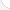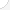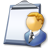StatisticiansDescription: Statisticians collect information from surveys and experiments. They decide where and how to gather the information, who to survey, and what questions to ask. They use the information they collect to make predictions about the economy or to assess various social problems. This helps business and government leaders make decisions.Source: Bureau of Labor Statistics, U.S. Department of Labor, Occupational Outlook Handbook, 2008-09 Edition at http://www.bls.gov/OCO/Complete Job Profile: http://www.bls.gov/oco/ocos045.htmSalary: \$50,001 or more per yearComments:Statisticians need calculus in college and must know all these topics to succeed in calculus. sormanic@member.ams.org christina sormani

There are 71 math topics Statisticians need to know.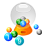Basic Math / Algebra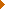FractionsDecimalsRatio and ProportionPercentCustomary MeasurementMetric MeasurementMeasurement ConversionBasic ProbabilityBasic StatisticsStatistical GraphingPowers and RootsOther Number BasesNegative NumbersScientific NotationBasic Problem Solving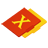First-Year AlgebraUsing FormulasLinear EquationsLinear InequalitiesOperations with PolynomialsFactoring PolynomialsRational ExpressionsCoordinate Graphing 2DLinear SystemsRadicalsQuadratic EquationsAlgebraic Representation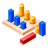GeometryBasic TerminologyAngle MeasurementCongruent TrianglesTriangle InequalitiesParallel LinesQuadrilateralsSimilarityGeometric MeanPythagorean TheoremRight Triangle TrigonometryCirclesConstructionsAreaVolumeTransformationsMake/Use 3D Drawings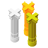Second-Year Algebra / TrigonometryFunctionsVariationImaginary NumbersPolynomial EquationsLogarithmsSequences and SeriesMatricesCoordinate Graphing 3DAdvanced ProbabilityAdvanced StatisticsConic SectionsNon-Linear SystemsTrigonometric/Circular FunctionsGraphs of Trigonometric FunctionsTrigonometric IdentitiesTrigonometric Equations/InversesOblique TrianglesPolar Coordinates/GraphsVectors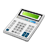Other TopicsCalculus and Higher MathBasic Calculator UseScientific Calculator UseComputer UseComputer ProgrammingGroup Problem SolvingMental MathInductive/Deductive ReasoningMath CommunicationsMathematical Modeling